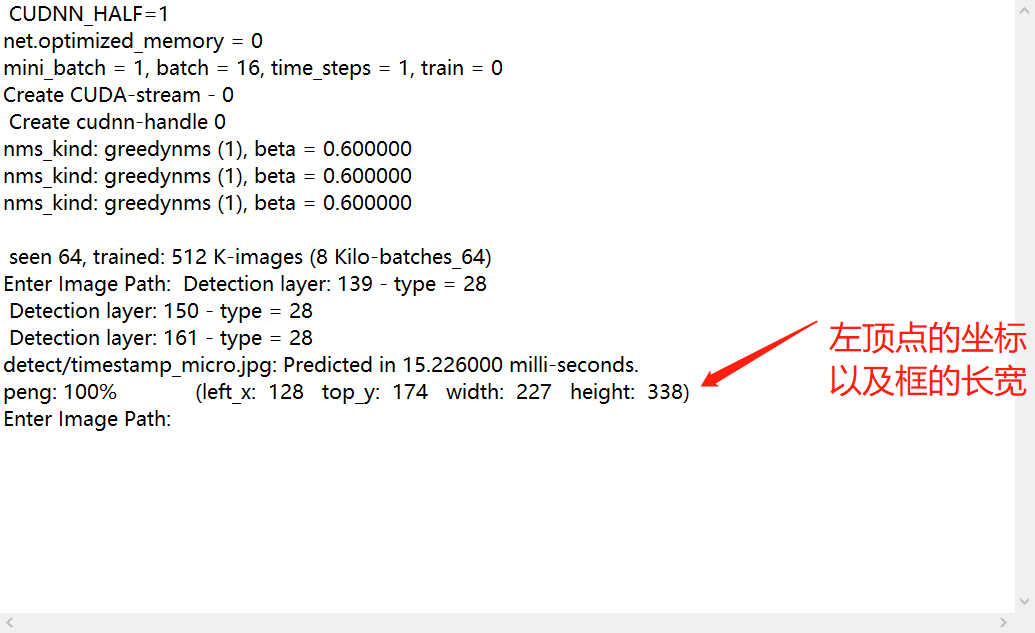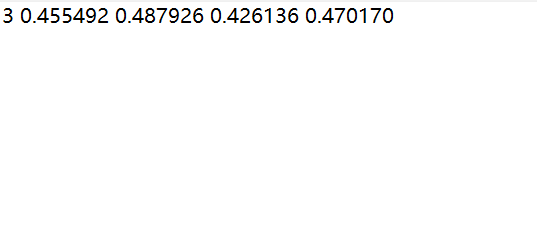2021-09-09 20:52

# yolov4生成的resul.txt是否能够提取数据转换成训练时所用的数据

yolov4检测完图片后会生成一个result.txt其中记录了照片中检测到目标的位置和大小yolov4训练时也需要使用LabeIimg标注训练照片，并也会生成相应的文本记录框选的位置和大小• 好问题 提建议
• 收藏

#### 1条回答默认 最新

•爱晚乏客游 2021-09-10 09:35
已采纳

可以的，但是你要先确定下生成的这个result的结果对不对，类别不对的话转成那个也没意义了。
这个可以直接转的

``````#size=（w,h）,box=[lrft_x,right_x,up_y,down_y],或者你也可以传入一个rect(wx,y,w,h)格式，然后替换掉函数里面的xywh
def convert(size, box):
dw = 1./(size)
dh = 1./(size)
x = (box + box)/2.0 - 1
y = (box + box)/2.0 - 1
w = box - box
h = box - box
x = x*dw
w = w*dw
y = y*dh
h = h*dh
if w>=1:
w=0.99999999
if h>=1:
h=0.99999999
return (x,y,w,h)

``````
已采纳该答案
评论
解决 无用
打赏 举报